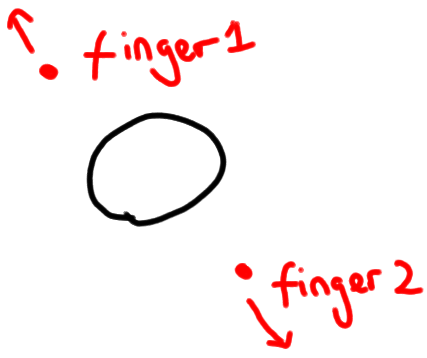# Scale Transform Axes (Non-uniform) With Pinch

Hi all,

I’m trying to scale a transform axes non-uniformly with pinch gesture. At the moment I’ve managed to do it uniformly by pinching anywhere on the screen, but I want it to scale in the x, y or z dependant on where you pinch around the gameobject.

So for instance if your fingers were to start diagonally to the gameobject and you were to pinch outwards the gameobject would increase scale both in the x and y (see image below) and visa-versa if you were to move them inwards. Futhermore, if your fingers were to start perfectly vertical to the gameobject it would only scale in the y axis.I’m guessing I need to get the dot product between the gameobject transform and the vector between finger 1 and 2 and based on that scale in the different axes, but I’m not sure if this is the best way to go about it. Note, we can disregard the z axis for this question.

Any help would be most appreciated.

Here’s my code so far (which does uniform scale):

``````if(Input.touchCount == 2)
{
float deltaMagnitudeDiff = 0f;

// Store both touches.
Touch touchZero = Input.GetTouch(0);
Touch touchOne = Input.GetTouch(1);

// Find the position in the previous frame of each touch.
Vector2 touchZeroPrevPos = touchZero.position - touchZero.deltaPosition;
Vector2 touchOnePrevPos = touchOne.position - touchOne.deltaPosition;

// Find the magnitude of the vector (the distance) between the touches in each frame.
float prevTouchDeltaMag = (touchZeroPrevPos - touchOnePrevPos).sqrMagnitude;
float touchDeltaMag = (touchZero.position - touchOne.position).sqrMagnitude;

deltaMagnitudeDiff = -(prevTouchDeltaMag - touchDeltaMag) * 0.1f;

transform.localScale = Vector3.Lerp(transform.localScale, transform.localScale * deltaMagnitudeDiff, Time.deltaTime);
}
``````

interesting.
i guess i would take the unit axis vector for each of XYZ (ie Vector3.Right, Vector3.Up, etc) and project those into 2D screenspace, and then DOT each of those vectors with your finger vector, and build a non-uniform scale in local-space with the results.

looks like unity doesn’t supply a routine for transforming a vector from local to screen-space,
but you could do it with Camera.WorldToScreenPoint if you also project a 4th point, the origin. (Vector3.Zero).

to account for the scaling which the axes vectors will undergo, i’d also consider uniformly scaling the 3 projected axes vectors so that the largest of them has unit-length. ie, find which one is longest, and scale all three of them by 1/magnitude(the longest one). you would do this before the dot-product.